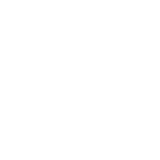Categories

# Introduction to Number System

## Objective Type Questions

### Fill in the blanks with the correct words.

1. he part of the computer system that you can touch and feel is the hardware.
2. A nibble is a group of 4 bits.
3. A computer stores information in the form of words.
4. 1KB is equivalent to 1024 bytes.
5. The hexadecimal number system includes letters A, B, C, D, E, and F.

### Write T for the true statement and F for the false one. Correct the false statement(s).

1. System software helps the user in performing a certain task. (F) (application software helps the user to perform a certain task)
2. A binary number can have only two digits, 0 and 1. (T)
3. The base of an octal number is 10. (F) (the base of an octal number is 8)
4. The hexadecimal number system has the base 16. (T)
5. The decimal number system consists of digits 0, 1, 2, 3, 4, 5, 6, 7, 8, and 9. (T)

### Choose the correct option.

Q1. Which of these is not an example of application software?
a. Payroll system
b. Fee collection system
d. Windows 7
Q2. 1GB is equivalent to:
a. 1024 KB
b. 1024 MB
c. 1024 TB
d. 1024 PB
Q3. Which of these is the binary equivalent of the decimal number 132?
a. 10000100
b. 10001000
c. 10010000
d. 10100000
Q4. Which of these is the equivalent decimal number of the binary number 11011.110?
a. 66.3
b. 33.6
c. 27.75
d. 36.6
Q5. Which of these is not a hexadecimal number?
a. ABGH
b. CD23
c. 12AB
d. EFCD

## Descriptive Type Questions

Q1. Differentiate between hardware and software.
Hardware comprises the physical components that make up a computer system. Software is a set of computer programs and procedures that provide instructions to the hardware about what to do and how to do it.
Q2. List the two categories of software with two examples each.
System software: operating systems, device drivers.
Application software: payroll system, inventory system.
Q3. What are the various storage units in the computer?
Bits, Byte, Nibble, Word, Kilobyte, Megabyte, Gigabyte, Terabyte, Petabyte, Exabyte, Zettabyte and Yottabyte.
Q4. What are the four number systems? Write their base values and digits used.
Binary number system has base 2 with digits 0, 1.
Octal number system has base 8 with digits 0, 1, 2, 3, 4, 5, 6, 7.
Decimal number system has base 10 with digits 0, 1, 2, 3, 4, 5, 6, 7, 8, 9.
Hexadecimal number system has base 16 with digits 0, 1, 2, 3, 4, 5, 6, 7, 8, 9, A, B, C, D, E, F.
Q5. List the steps to convert a binary number to its equivalent base 10 (decimal) number.
Step 1: Find the positional value of each digit.
Step 2: Multiply the digit with the positional value.
Step 3: Sum up the product calculated in step 2.
Step 4: The total is the equivalent value in the decimal number system.

## Extra Questions.

Q1. The computer system comprises hardware and software.
Q2. The symbols 0 and 1 are together called binary digits or bits.
Q3. What is system software?
It is a set of one or more programs that is designed to control and manage the operation of a computer system.
Q4. What is application software?
It comprises computer programs that are designed to help the user in performing a certain type of work.
Q5. Bit is the smallest data unit in a computer.